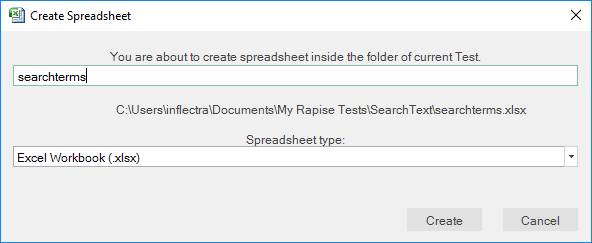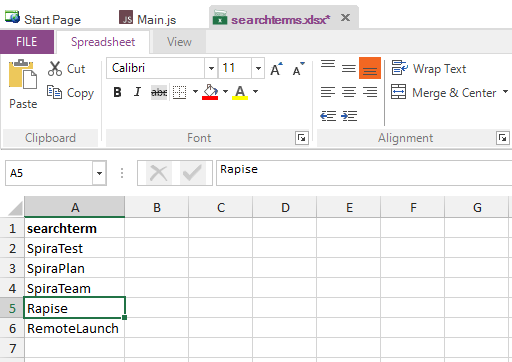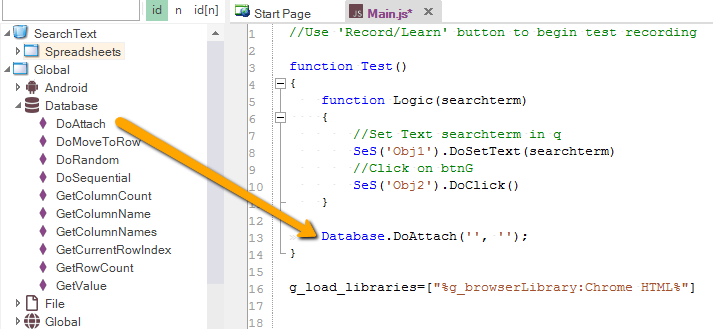# Data-Driven Testing (JavaScript)

## Purpose

Data Driven Testing is an automated testing technique in which test case data is separated from test case logic.  Each set of test case data consists of input values and a set of expected output values.  The actual output values are compared to the expected output values to determine whether the test passed.

You can perform data-driven testing either using an MS-Excel spreadsheet as the datasource or a relational database.

The Spreadsheet object is useful for implementing data-driven tests. It allows you to connect to, query, and read an excel spreadsheet from your test script.  To create a data-driven test, you will:

### 1. Record a Test

The exact inputs you use for the recording will not matter as much as your interactions with the objects.  The following excerpt was recorded using www.google.com:

``````    function Test()
{
//Set Text Inflectra in q
SeS('Obj1').DoSetText("Inflectra");
//Click on btnG
SeS('Obj2').DoClick();
}
``````

The actions recorded were: (1) Type Inflectra into the search box. (2) Press the Google Search button.

### 2. Parameterize the Test() Function

The `Test()` function has all of the procedural logic for the test.  Replace input values with variables.  Encapsulate the logic in a nested function with one parameter for each variable you created.  As an example, we will parameterize the `Test()` function we created in step one:

``````function Test()
{
function Logic(searchterm)
{
//our new function encapsulates the test logic
//Set Text using searchterm
SeS('Obj1').DoSetText(searchterm) //here we changed a hard-coded value into a variable
//Click on btnG
SeS('Obj2').DoClick()
}
Logic("Inflectra") //don't forget to call your new function
}
``````

### 3. Create the Test Case Data

Create a spreadsheet with name searchterms.xlsx. For this right click the test node and choose `Create > Spreadsheet...`.Enter the name and click `Create` button.In the spreadsheet create a column for every variable in step two.  Add columns for any expected output values you wish to verify. Each row is a test case. In our google example, we only have one input value (`searchterm`) and we're not comparing any expected output values, so we will only need one column in our spreadsheet.### 4. Attach Spreadsheet Object to searchterms.xlsx

Drag the searchterms.xlsx from files tree into appropriate place in your test source:### 5. Use Spreadsheet to Access the Test Case Data

In our example, we use a Spreadsheet object and run the test logic once for every row.

``````function Test()
{
function Logic(searchterm)
{
//Set Text searchterm in q
SeS('Obj1').DoSetText(searchterm)
//Click on btnG
SeS('Obj2').DoClick()
}

// Go through all rows
{
// Read cell value from column 0
// Pass it into Logic function
Logic(term);
}
}
``````

## Using a Relational Database

Rapise comes with the Database query global object that allows you to send SQL queries to a database and then iterate through the results. The process for creating such a data-driven test is as follows:

### 1. Record a Test

The exact inputs you use for the recording will not matter as much as your interactions with the objects.  The following excerpt was recorded using www.google.com:

``````    function Test()
{
//Set Text Inflectra in q
SeS('Obj1').DoSetText("Inflectra");
//Click on btnG
SeS('Obj2').DoClick();
}
``````

The actions recorded were: (1) Type Inflectra into the search box. (2) Press the Google Search button.

### 2. Parameterize the Test() Function

The `Test()` function has all of the procedural logic for the test.  Replace input values with variables.  Encapsulate the logic in a nested function with one parameter for each variable you created.  As an example, we will parameterize  the `Test()` function we created in step one:

``````function Test()
{
function Logic(searchterm)
{
//our new function encapsulates the test logic
//Set Text using searchterm
SeS('Obj1').DoSetText(searchterm) //here we changed a hard-coded value into a variable
//Click on btnG
SeS('Obj2').DoClick()
}
Logic("Inflectra") //don't forget to call your new function
}
``````

### 3. Use Database to Connect the Test Case Data.

This assumes that you already have an ODBC or OLE DB compatible relational database that contains the necessary test data. You drag the `Database` global object into the script editor:and then use:

• Database.DoAttach() - to make the database connection and specify the SQL query
• Database.GetRowCount() - to verify that there is data
• Database.DoSequential() - to loop through the data set row by row
• Database.GetValue() - to get that row's data

Here is an example of the code needed to loop through a list of records (taken from the SpiraTest database as an example) and call our `Logic()` parameterized function with the appropriate test data:

``````var success = Database.DoAttach('Provider=SQLOLEDB.1;Integrated Security=SSPI;Persist Security Info=False;Initial Catalog=SpiraTest;Data Source=.' , 'SELECT * FROM TST_PROJECT' );
Tester.Assert('Successfully Connected', success);

var count = Database.GetRowCount();
Tester.Message(count);

//Loop through the rows
while(Database.DoSequential())
{
var projectId = Database.GetValue("PROJECT_ID" );
var name = Database.GetValue( "NAME");
var description = Database.GetValue("DESCRIPTION" );
Logic(name);
}
``````